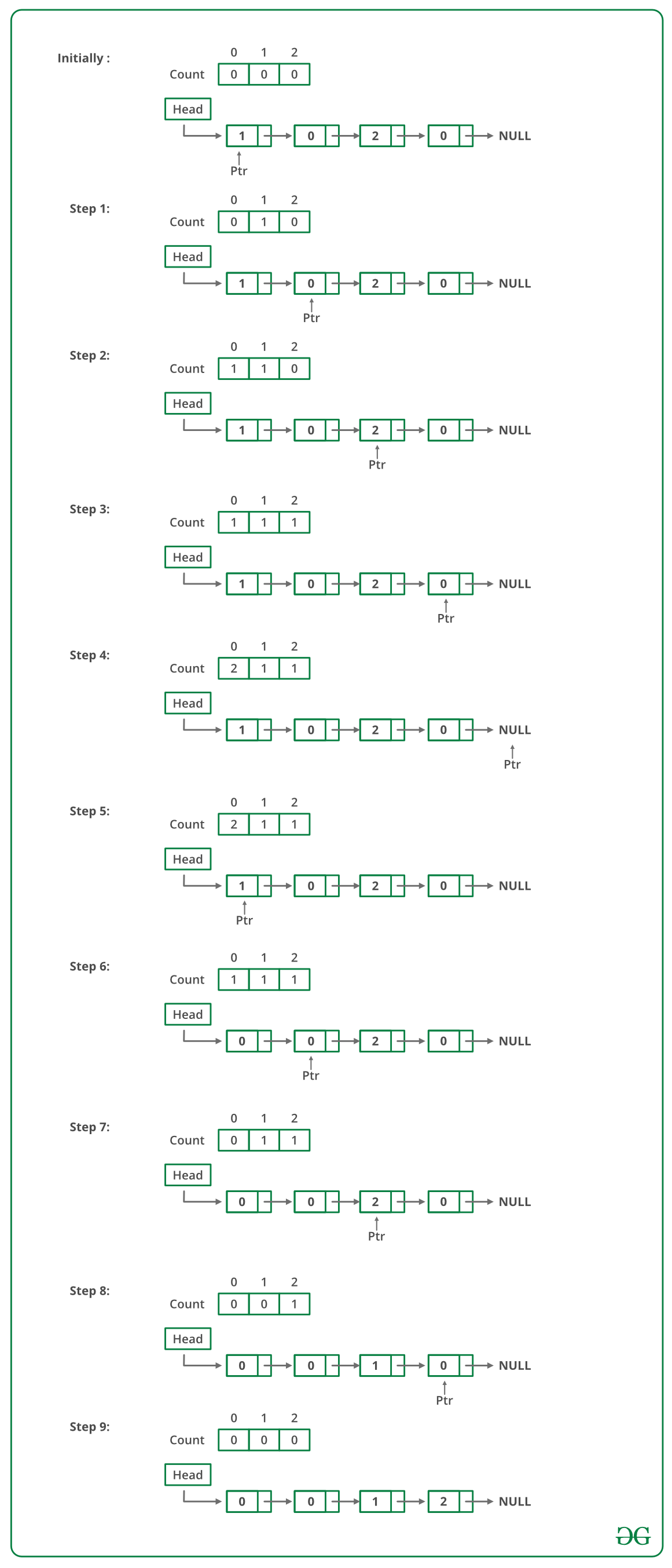# Java Program For Sorting A Linked List Of 0s, 1s And 2s

• Last Updated : 22 Dec, 2021

Given a linked list of 0s, 1s and 2s, sort it.
Examples:

```Input: 1 -> 1 -> 2 -> 0 -> 2 -> 0 -> 1 -> NULL
Output: 0 -> 0 -> 1 -> 1 -> 1 -> 2 -> 2 -> NULL

Input: 1 -> 1 -> 2 -> 1 -> 0 -> NULL
Output: 0 -> 1 -> 1 -> 1 -> 2 -> NULL
```

Source: Microsoft Interview | Set 1

Following steps can be used to sort the given linked list.

• Traverse the list and count the number of 0s, 1s, and 2s. Let the counts be n1, n2, and n3 respectively.
• Traverse the list again, fill the first n1 nodes with 0, then n2 nodes with 1, and finally n3 nodes with 2.

Below image is a dry run of the above approach:Below is the implementation of the above approach:

## Java

 `// Java program to sort a linked list ``// of 0, 1 and 2``class` `LinkedList``{``    ``// Head of list``    ``Node head;  ``  ` `    ``// Linked list Node``    ``class` `Node``    ``{``        ``int` `data;``        ``Node next;``        ``Node(``int` `d) ``        ``{``            ``data = d; ``            ``next = ``null``; ``        ``}``    ``}`` ` `    ``void` `sortList()``    ``{``       ``// Initialise count of 0 1 ``       ``// and 2 as 0``       ``int` `count[] = {``0``, ``0``, ``0``}; ``        ` `       ``Node ptr = head;``        ` `       ``/* Count total number of '0', '1' and '2'``          ``count will store total number of '0's``          ``count will store total number of '1's``          ``count will store total number of '2's  */``       ``while` `(ptr != ``null``) ``       ``{``            ``count[ptr.data]++;``            ``ptr = ptr.next;``       ``}`` ` `       ``int` `i = ``0``;``       ``ptr = head;`` ` `       ``/* Let say count = n1, count = n2 ``          ``and count = n3``          ``now start traversing list from head node,``          ``1) fill the list with 0, till n1 > 0``          ``2) fill the list with 1, till n2 > 0``          ``3) fill the list with 2, till n3 > 0  */``        ``while` `(ptr != ``null``) ``        ``{``            ``if` `(count[i] == ``0``)``                ``i++;``            ``else` `            ``{``               ``ptr.data= i;``               ``--count[i];``               ``ptr = ptr.next;``            ``}``         ``}``    ``}                 ``                    ` `    ``// Utility functions ``    ``/* Inserts a new Node at front ``       ``of the list. */``    ``public` `void` `push(``int` `new_data)``    ``{``        ``/* 1 & 2: Allocate the Node &``                  ``Put in the data*/``        ``Node new_node = ``new` `Node(new_data);``  ` `        ``// 3. Make next of new Node as head ``        ``new_node.next = head;``  ` `        ``// 4. Move the head to point to ``        ``// new Node ``        ``head = new_node;``    ``}`` ` `    ``// Function to print linked list ``    ``void` `printList()``    ``{``        ``Node temp = head;``        ``while` `(temp != ``null``)``        ``{``           ``System.out.print(temp.data + ``" "``);``           ``temp = temp.next;``        ``}  ``        ``System.out.println();``    ``}`` ` `    ``// Driver code``    ``public` `static` `void` `main(String args[])``    ``{``        ``LinkedList llist = ``new` `LinkedList();``         ` `        ``/* Constructed Linked List is ``           ``1->2->3->4->5->6->7->8->8->9->null */``        ``llist.push(``0``);``        ``llist.push(``1``);``        ``llist.push(``0``);``        ``llist.push(``2``);``        ``llist.push(``1``);``        ``llist.push(``1``);``        ``llist.push(``2``);``        ``llist.push(``1``);``        ``llist.push(``2``);``         ` `        ``System.out.println(``               ``"Linked List before sorting"``);``        ``llist.printList();``         ` `        ``llist.sortList();`` ` `        ``System.out.println(``               ``"Linked List after sorting"``);``        ``llist.printList();``    ``}``} ``// This code is contributed by Rajat Mishra `

Output:

```Linked List Before Sorting
2  1  2  1  1  2  0  1  0